# Lambert Cosine Emission¶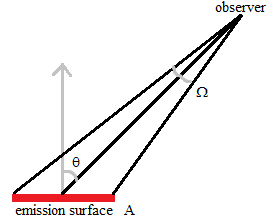Fig. 26 Emission surface observed from angle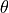relative to surface normal, and solid angle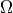.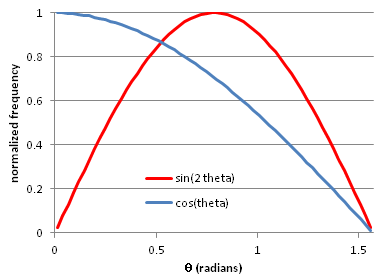Fig. 27 Normalized observed current per solid angle (blue) and emitted current per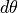(red) v.s..

A Lambertian emitter has the same brightness (i.e. current per area per solid angle) when observed from all angles. A Lambertian emitter therefore follows Lambert’s cosine law, which is to say that the angular current density (i.e. current per solid angle) observed from an angle(relative to the surface normal) is proportional to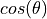. That is because the emission surface appears to have a size proportional to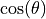from a vantage point of that angle. The angular current density is independent of the angle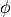of rotation around the surface normal.

FLY2: See SIMION Example: particles (lambert_cosine.fly2) (added in 2015-07-15) for an example of generating a Lambert Cosine distribution of emission in a Monte Carlo Method fashion in a FLY2 file.

Sampling: If all rays traced in a beam represent the same current, we can sample a random ray from a Lambert cosine distribution in Monte Carlo fashion as follows. The probability of a ray with angleis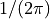for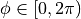. This gives a random variable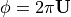, where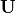is a uniformly distributed random variable between 0 and 1. The probability of a sampling a ray with angleis proportional not tobut to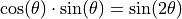since the solid angle covered by all observers (at any) with angleis proportional to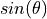. Inverting this distribution,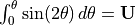, implies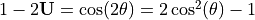. So, we have the random variable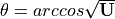.